## 往年例题

### 填空

1. 为评估函数$f$的近似值$h$，常用的评价标准包括————，————和完备性；而因为计算的复杂性，我们无法计算$h$在所有数据上的误差，因此采用$h$在————上的平均误差来近似。
2. 函数$y=f(x_1,x_2,…,x_n)$的所有自变量都是二值的，即$x_i\in {0,1}$，且$y\in {-1,0,1}$，这样不同的函数$f$有————个；监督学习问题的实例是指由某个特定的标记样本集所代表的学习问题，现在若仅知道标记样本训练集包括$m\le2^n$个数据，这样的问题实例有————个，$f$的近似值$h$的候选项可能有————种；$f$的最简单近似值$h$包括————个标量函数。
3. 策略$\pi$的价值$V_\pi(s)$中$s$的意思是————，Q值$Q(\pi, s, a)$的意思是指————；强化学习中基于模型的方法，就是为了获得对————的描述。
4. 逻辑的三要素是： ————，————，————
5. 博弈的基本要素是————，—————，————，纳什均衡指————
6. 常见的激活函数有：————，————，————
7. 解释性较好的分类算法有————和————

### 问答

8). 搜索，如下图的所示状态图，不同节点代表不同的状态，假设所有边上的路径耗散都为单位1，初始状态为J，目标状态为D，回答问题：

• 用宽度优先搜索算法，找出从J到D的最短路径，共多少次访问节点？
• 用IDS算法，找到从J到D的最短路径，共多少次访问节点？
• 若状态D和K之间连一条边，采用IDS算法，找到J到D的最短路径，共多少次访问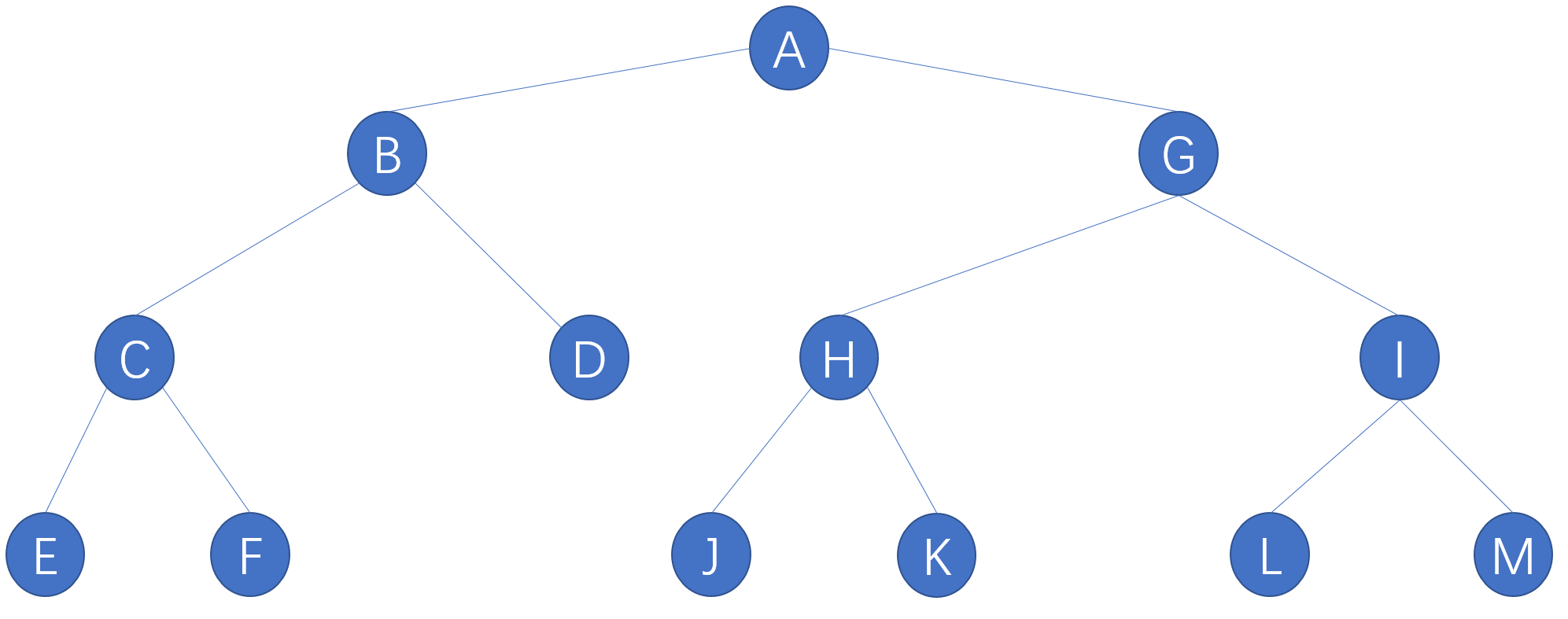9) 启发式搜索 假设有N个红军和N个蓝军，船每次最多可供K个人乘渡，这里$N=5,K\le3$。假设在某个时刻，在河的左岸有M个红军，C个蓝军，B=1表示船在左岸，B=0表示船在右岸。现在以它们的组合为状态$n=(M,C,B)$的描述。给定下面的两种不同的启发式函数：

1. $h_1(n)=M+C$
2. $h_2(n)=M+C-2B$
请说明$h_1(n)$不满足$h_1(n)\le h^*(n)$而$h_2(n)$满足

10) 概念题： 谈谈你对搜索问题，MDP，强化学习，完美信息下的动态博弈和非完美信息下的动态博弈的理解，分析它们之间的异同。

11) 概念题： 简述过拟合产生的原因，通过修改损失函数可以避免过拟合，举两个例子说明这种方法。

12) 给定如下图的神经网络，且$\sigma(z)=(1+e^{-z})^{-1},h_j=\sigma(v_2\cdot \phi(x)),score=w\cdot [h_1,h_2]$,回答下面的问题：

• 若采用损失函数$loss_{hinge}(x,y,W)=max(1-W\cdot \phi(x)y,0)$来评估一个样本的准确性，画出相应的梯度/偏导计算图
• 若在输出层的误差是单位1，权值$V$该如何调整？假设步长/学习率为$\lambda_0$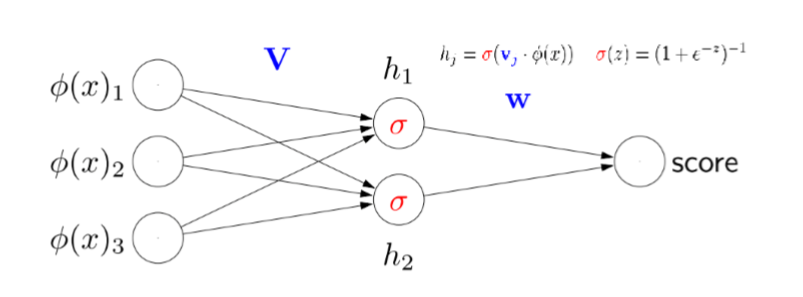13) Bayes网络 给定Bayes网络结构如下图，其中$E$是观测变量$H$是隐变量，回答问题：

• 请由网络结构给出联合概率密度的乘积计算公式
• 若该联合概率质量函数用一表格完全描述，表格有多少行（用记号$|\cdot|$表示集合大小）
• 用Bayes网络结构和一些条件概率表可以约简上述联合概率质量函数，这些条件概率表（包括边缘概率表）共有多少行？
• 若该联合概率密度函数用一表格完全描述，请用SQL语句或伪代码描述求概率$p(H_2=a|E_1=e_1,E_4=e_4)$的过程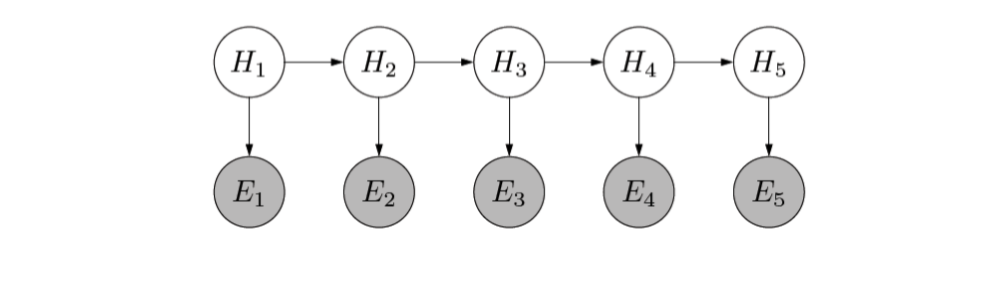14) 搜索： 如下图所示的状态图，I是初始状态，G是目标状态，假设每个状态至多被访问一次

• 采用宽度优先，写出节点访问次序，访问节点的数量或数量范围是多少？
• 设计启发式函数$h(N)=$“节点N和目标节点G之间所有路径的最小边数”，判断该启发式函数是否可采纳，并给出理由
• 采用A*算法解决本搜索问题，节点搜索次序是什么，这些被搜索节点的$f$值是多少？（若上一小题中启发式函数是可采纳的，就用该启发式函数，否则，请设计一个新的可采纳启发式函数）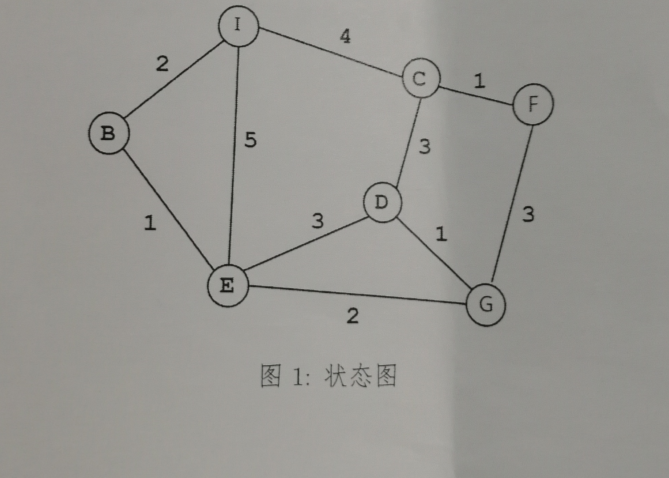15) 岭回归问题。 求解线性回归问题是，$\hat{W}=argmin_{W}{|y-XW|^2 + \lambda |W|^2 }$。 其中$X\in \mathbb{R}^{n\times d}$是$n$个$d$维的数据,$X$每列和为0，且$rank(X)=d,y\in\mathbb{R}^n$，$W\in R^n$是参数向量。岭回归问题就是寻找最优参数$\hat{W}$使得损失函数和$L_2$正则项的线性组合构成的目标函数最小。 请用矩阵运算，给出最优解$\hat{W}$的计算过程和结果。

16) 赛马时，一线人会在赛前告诉你某匹马Belle没吃早餐。假设：

• 马输赢取决于其健康和速度，而健康和速度无关。
• 健康的马比病马吃早餐的概率大
• 线人情报的可靠性不是100%
求解下列问题：
• 画出5个变量的贝叶斯网络，其中T:得到情报，B:Belle吃早餐，H:Belle健康，W:Belle赢，F:Belle很快
• 根据贝叶斯网络结构，给出联合概率$p(T,B,H,W,F)$的乘积计算公式。给出边缘概率$p(W)$的计算公式
• 计算条件概率$p(W|T)$，即依据线人情报来计算Belle获胜的概率

17) 学习问题：

• 写出线性回归和逻辑斯蒂回归的目标函数
• 写出SVM的目标函数
• 写出加入松弛变量后的SVM的优化目标

### 参考答案

1. 准确性 复杂性 验证集
2. $3^{2^n}$ , $3^{C^m_{2^n}}$ , $3^m$ , $n+1$
3. 状态，从状态$s$出发采取行动$a$后继续采用策略$\pi$的收益
4. 语法，语义，推理规则
5. 参与者，策略集，收益，参与者们选择的策略互为最佳应对
6. Sigmoid，tanh，ReLu
7. KNN，决策树
8. （1）11 （2）迭代深入搜索，访问34次节点（3）14
9. hint: 证明$h_1$不满足条件举个反例就行，例如状态为$(1,1,1)$时$h_2=2$而实际上摆渡一次就能到达目标状态。
证明$h_2$满足条件可以分情况讨论（船在左岸和右岸），可以参考“传教士和野人渡河”的问题解法。

10. 概念题，看ppt即可。

11. 概念题，同上。
12. （1）参见第13份ppt第22页。 （2）将误差（即残差）到$V$的偏导计算图上边的权值连乘再乘上$\lambda_0$即可
13. （1） $p(E1,…E_n,H_1,…H_n)=p(H_1)\prod{i=2}^5p(Hi|H{i-1})\prod{i=1}^5p(E_i|H_i)$ （2） $\prod_i|H_i||E_i|$ （3）$|H_1|+\sum{i=2}^5|Hi||H_i-1| +\sum{i=1}^5 |Ei||H{i-1}|$ （4）可以参考本篇笔记中chap13的内容
14. （1） I->BEC->DGF 共7个节点 （2）是可采纳的。设N到G的最优路径的每条边权值为$p_i$共$k$条边，由于$p_i\ge 1$，则$\sum p_i\ge k$，由此：$h(N)\le h^*(N)$，得证 （3）I->B->E->G
15. 将原式求导为0可得。 （注意这里题目中维度描述有错误）

16.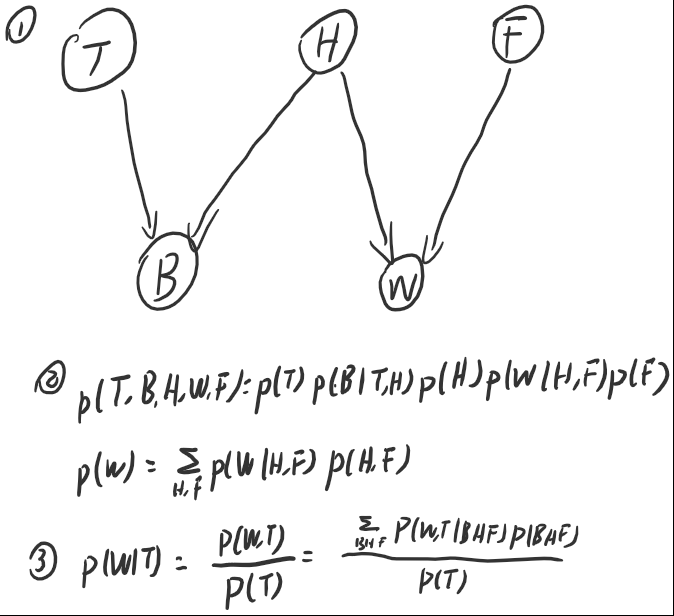17. 很简单了，ppt里都能找到

## Chap3 问题求解：搜索

• 状态空间
• 后继函数
• 初始状态
• 目标测试
• 路径耗散

### 搜索问题举例

• 状态空间， 期盼任意一种布局
• 后继函数： 合法移动棋子得到另一种布局
• 初态：任何初始布局，终态：曹操跑出来
• 路径耗散：每移动一次棋子，耗散为1
• 解： 从初态到终态的移动序列；最优解：路径耗散最少的序列

### 五要素

• 尽量覆盖所有合法状态，并尽可能让状态都是可达，可行合法的。
• 状态数目越少越好。
• 方便设计后继函数和搜索算法。

• 某个精准的特定状态
• 满足某些条件的某个状态
• 满足某些条件的状态机和

• 网格化
• 扫描线方法
• 障碍物边界法

## Chap4 盲搜索

• 分支因子$b$： 后继函数返回的最大状态数目
• 从初态到目标状态的最小深度$d$：搜索树上离根最近的目标节点的深度

• 完备的
• 每条边的路径耗散相等时满足最优性，否则不一定
• 复杂度：$O(b^d)$

• 对于优先搜索树完备
• 不具备最优性
• 时间复杂度$O(b^m)$，空间复杂度$O(bm)$，$m$为叶子节点的最大深度

• 每次扩展时只扩展一个节点，节省内存
• 可能不完备，也可能得不到最优解

• 完备的
• 边的路径耗散相同时满足最优，否则不一定
• 时间复杂度$O(b^d)$，空间复杂度$O(bd)$

### 避免重复访问

• 设置标识数组，标记状态是否被访问过
• 用Open/Closed表，Closed表存储所有访问过的状态，未拓展的状态用Open来表示，若当前待扩展的转台在Closed中，将其丢弃，否则扩展

### 完备性

• 状态空间无限，一般不完备
• 状态空间有限，但是每个状态允许被无限次访问，搜索不完备
• 状态空间有限，访问重复的节点被丢弃，则搜索完备，但一般不是最优

## Chap5 启发式搜索

1. $f(N)=g(N)+h(N)$ 完整解的路径耗散，对应A*算法
2. $f(N)=h(N)$从当前节点到目标节点的路径耗散，对应贪婪算法

$N$：待评价的节点，$g(N)$从初始节点到$N$的路径耗散，$h(N)$从$N$到目标节点的路径耗散，就是所谓启发式函数
$g(N)$: 从初始节点到N的路径耗散
$h(N)$: 从N到目标节点的路径耗散，即所谓启发式函数

• $h(N)\ge 0,h(Goal)=0$且$h(N)$越小离目标越近（基本要求）。
• 可采纳 （admissiable）启发式函数：假设$h^(N)$是节点N到目标节点的实际最优路径耗散，那么当$0\le h(N)\le h^(N)$时启发式函数是可采纳的，其核心观点是“乐观”地估计路径耗散。
• 一个比可采纳更强的性质是一致的（单调的）:当$h(N)\le c(N,N’)+h(N’)$时，$h$是一致/单调的，其中$N’$为后继节点，$c(N,N’)$为两点之间的单步耗散

$f(N)=h(N)=\text{Manhattan}\ \text{distance}$ 启发式函数为从当前点到终点的曼哈顿距离。

• 错误放置的格子数（可采纳，一致的）
• 所有数字到其正确位置的曼哈顿举例之和（可采纳一致的）
• 逆序数之和（不可采纳，证明：举例反证）

• 欧式距离（可采纳的，一致的）
• 曼哈顿距离（若不允许沿网格对角线移动，则是可采纳的一致的；否则是不可采纳的不一致）

### A*算法

$f(N)=g(N)+h(N)$ 完整解的路径耗散，对应A*算法
$g(N)$是初始节点到N的路径耗散，$h(N)$是从N到目标节点的路径耗散，可采纳的启发式函数。

1. 状态空间无限/允许重复访问， A*算法的搜索不会停止
2. 求解实际问题时通常给一个停止时间，到达时间时自动停止

A*算法状态重复访问改进：当且仅当到该节点的新路径的耗散$g(N)$比以前访问该状态的路径耗散更大时，丢弃重复访问的这个状态节点。

A算法中一致的启发式函数：

• 初始化$f$的阈值$t$为$f(N0)$
• Loop:
• 执行深度优先搜索，扩展$f(N)\le t$的节点
• 重新设置$t$为未拓展节点中$f$的最小值

• 完备+最优
• 内存要求比A*少
• 避免了未拓展节点集合的排序开销

• 无法充分利用内存，两次迭代之键只保留阈值$t$
• 无法避免重复访问不在路径上的点

## Chap6 优化问题

$n$元实函数$g(x)$在$n$维空间中变化速度最快的方向$\nabla_xg=(\frac{\partial g}{\partial x_1},\frac{\partial g}{\partial x_2},\frac{\partial g}{\partial x_3}…,\frac{\partial g}{\partial x_n})$

• 第一步，设置超参数学习率（步长）
• 第二步，初始化初态
• 第三部，循环迭代

• 不能保证全局最小
• 停止条件和学习率设置需要人工经验

• 不知道步长时就多试几个步长来尝试
• 随机梯度下降，每一步都更新参数，而不是处理完整个训练集后更新

• best-found 选择目标函数最小的邻点
• first-found 随机选择一个（比当前点）更好的邻点
• Random Walk 随机选择一个邻点（即使其目标函数大于当前点）

• best-found 离散版本的梯度下降
• first-found 随机梯度下降

• 用不同随机初始值重启算法
• 在局部最优点增加扰动调整邻居算子
• 模拟退火（爬山+随机行走）

LBS 局部剪枝搜索

• 假设每个解有$l$个后继，每步都在所有$lk$个后继中选择最优的$k$作为当前的$k$个解
• 所有$lk$个后继对应一个被选择的概率分布，

$T$是温度，在整个搜索过程中温度会不断下降，理论可以证明，T下降的足够慢，那么算法找到最优解的概率逼近1.

Tabu搜索/禁忌搜索

• 维护一个长度有限(设为$k$)的最近历史解队列$\text{Tabu}_k$
• 搜索过程中，发现新解出现在$\text{Tabu}_k$中，直接放弃
• $\text{Tabu}_k$能够在一定长度下防止陷入局部最优

(1+1)EA 进化算法/遗传算法

EA算法的后继函数可以：

• 从当前解集的一个解产生后继，类似无性繁殖
• 从当前解集的两个或多个解产生后继，类似于有性繁殖

• 在当前k个解中，随机选择两个产生孩子，这称为交叉算子，这也是一般的进化算法
• 在当前k个解中，选择3个计算后继为$x=a+\zeta(b-c)$，得到差分演化算法
• 在当前k个解中，全部选择，用来估计一个分布，以用来描述整个解集。解集获得的过程我们称为从该模型中进行采样，这就是估计分布算法 EDA
• 在当前k个解中寻找后继时，考虑k个解的搜索历史，找到每个解历史最佳解和k个历史最佳解，以这些解为基础，设计速度函数，获得k个解的后继，即粒子群算法
• 在当前k个解中，每个解的后继都是以该解为初始解，执行局部搜索（例如使用爬山法），得到局部极值，然后以此局部极值解为后继，即memetic算法
• 在状态空间的设计/编码上引入交叉/变异/选择算子，即遗传算法

## Chap7 CSP 约束满足问题

$\text{CSP}=(X,D,C)$是一个三元组：

• $X$为一组变量，不妨设为一个n维向量
• $D$为值域，定义了每个变量的值域
• $C$是一个约束组，是$m$个计算公式，约束X分量之间取值的关系。
$X_i$从$D_i$中取值，所有变量都给一个取值，形成一个赋值$v=(x_1,x_2,…,x_n)$可以参与约束$C$的计算，判断约束是否被满足

8个变量，约束条件分为两类1.每行一个皇后，2.每个对角线一个皇后。

• 状态：有效赋值
• 初始状态： {}， k=0
• 后继函数： 在未赋值变量中挑选一个为第k+1个变量并对第k+1个变量赋值
• 目标测试：k=n
• 路径耗散： 假设单步耗散为1

CSP问题的特性在于可交换性

• 扩展节点时可以任意选择一个未赋值的变量来扩展节点
• 不需要存储到达当前节点的路径，因此可以用回溯算法

1. 前向检验：提前检测出冲突，以8皇后为例，一旦赋值了某个变量，那么按照约束也就可以把其他变量的某些取值从值域中去掉。
2. 启发式地选择下一个赋值的变量，选择约束最强/残留值域最小的变量，使得分支因子最小，后继最少
3. 给残存的值域排序，对不同的v做前向检验
4. 约束传播（课后了解）

## Chap8 机器学习

• 准确性 即$h,g$之间的近似度
• 复杂性 $h$的描述长度，应保证在合理有效范围内以得到可满足的时空代价
• 完备性 $h$是否对$f$的每个输入都定义了一个响应

## Chap9 线性回归

• 绝对损失 $|f(x)-h(x)|$
• 平方损失 $(f(x)-h(x))^2$
• 计数损失 $1*(f(x)\neq h(x))$

• 采用平方损失函数：近似寻找均值，综合所有样本影响
• 采用绝对损失函数：近似寻找中值，抵抗离群点影响

• 平方损失函数时可以用最小二乘法
• 梯度下降法

## Chap10 线性分类

### SVM

• 约束条件 找到一个分类面$h(x)$，它能够分开所有的训练数据，并是的任意一个数据点到分类面的距离（几何间隔）大于等于$\frac{1}{|W|}$
• 优化目标 $\frac{1}{|W|}$越大越好
• 这里优化问题来自对分类问题的几何意义的理解和形式化，该问题追求“结构风险最小化” 这不同于我们之前定义的训练误差最小化和经验风险最小化

### SMO 算法

• 每次固定m-2个乘法因子$\alpha_i$让其他两个任意变化，寻找者两个任意变化乘法因子的最佳值，循环迭代调整固定不同的 m−2 个乘 法因子 α，直到收敛。
• 局部搜索去寻找自由变量$\alpha_i$就是对应的支持向量机器非零的$\alpha_i$
• 训练过程中，尽量避免每次循环迭代都扫描一遍训练数据（因此不适合海量数据）

## Chap12 非线性处理

### 决策树

• 初始时刻构建树根，拥有所有训练数据
• 对每个节点，选择数据的某属性来分裂剩余数据集
• 当某节点的所有训练数据都属于同一类时划分结束，生成叶节点

$H(N)=-\sum_ip_ilog(p_i)$
$p_i$是节点$N$中拥有数据集$D$中属于第i类数据在D中占比。

Gini指数

## Chap13 神经网络

### 特征提取函数

• 定义输入$x$的各个单项式构成的向量为特征$\phi(x)$
• 存在问题： 单项式的最高次数难以确定 （高了过拟合/低了欠拟合）

• $W,\phi(x)$都是值向量，它们做内积运算得到分数score，是线性运算
• 非线性部分，可以用非线性函数获得

ReLu的优点：

• 稀疏激活性： 只激活少量神经元
• 单侧抑制和相对宽阔的兴奋边界：降低梯度消失的影响

## Chap14 贝叶斯网络

• 专家建模
• 从数据集中自动学习
• 二者结合

• 统计频率到边缘概率$p(T)=(0.6,0.4)$
• 令 $T=c,s$时分别统计各个打分的频率得到条件概率
$p(R|T)=((0,0,0,0,0.67,0.33),(0.5,0,0,0,0.5))$

\pi$不同的是，在状态$s$是采用了不同行动，即$Q\pi(s,a)$是$\pi,s,a$的函数，而$V\pi(s)$只是$\pi,s$的函数 策略改进算法： • 输入策略$\pi$，得到一个更好的策略，冲锋利用马尔可夫性 • 对任意状态$s$，执行下述操作 • 对不同行动$a$计算$Q_\pi(s,a)$• 更新$\pi{new}=argmax{a}Q_\pi(s,a)$类似爬山法，把状态s所有可能行动都尝试一遍。 结合策略评估和策略改进，我们得到值迭代算法来计算最优策略： • 对所有状态 s 初始化 V0opt(s) ← 0; • for t = 1,2,…,T0 次: • 对每一个状态 s，执行： •$V^t{opt}(s) ←max a \in Action(s)\sum s’ T(s,a,s’)[Reward(s,a,s’) +V^{t-1}{opt}(s’)]$折扣因子：我们再计算路径收益时，对未经历的路径的收益乘一个折扣因子$\lambda$，离现在越远，收益打折强度越大。 那么递推方程改为:$V\pi(s) =\sum{s’\in S}T(s,a,s’)[U(s,a,s’) +\lambda V_\pi(s’)] $## Chap16 强化学习 因为在现实生活中，我们通常不知道状态转移概率和奖励的细节，因此MDP有很大的局限性，强化学习由此而生。 MDP 强化学习 转移概率和奖励情况已知，离线 无人知道所有转移概率和奖励，在线 决策判断都可以仿真 需要花费代价去搜索未知（转移概率和奖励）$f$已知$f$未知 强化学习算法框架： • for t=1,2,… • 选择行动$at=\pi(s{t-1})$• 收集反馈奖励$r_t$，获得新状态$s_t$• 更新参数 已知：$s_0,a_1,r_1,s_1,a_2,r_2,…,a_n,r_n,s_n$求：$T(s,a,s’),U(s,a,s’)$蒙特卡洛方法 • 从已知数据任何状态开始$(s,a,r,s’)$被视为一组数据，源数据序列被分割为n段 • 计算$\hat{T}(s,a,s’)=\frac{ M(s,a,s’)}{M(s,a)},\hat{U}(s,a,s’)=\frac{\sum_r(s,a,r,s’)^r}{M(s,a,s’)}$• 用$\hat{T}$近似$T$，$\hat{U}$近似$U$这类方法被称为基于模型的蒙特卡洛算法。它们对数据量要求大，还要求样本数据满足独立性假设。 模型无关方法 即不去揣摩状态转移函数和奖励，直接评估策略。 定义引入折扣因子的时刻t的收益：$ut=r_t+\lambda r{t+1}+\lambda^2r{t+2}+\cdots$然后用$u_t$的均值当成$Q
\pi(s,a)$算法描述： • 对任意时刻$t$，对应数据段$(s,a,r)$: • 计算$(s,a,u_t)$• 令$\xi=$1/ (更新次数+1) • 更新$Q\pi(s,a)=(1-\xi) Q\pi(s,a)+\xi u_t$求最优策略时第一种方法类似于MDP，第二种方法就是Q学习。 ## Chap17 博弈与对抗性搜索 博弈的基本要素： • 参与者： 大于等于2 • 策略集：可采取的行动的集合 • 收益：$P_i(a_1j_1,…,a_nj_n)$表示参与者在所有人策略为$(a_1j_1,…,a_nj_n)$时的收益 ### 静态博弈 完美信息：每个参与者对博弈信息完美了解 静态博弈：所有参与者同时选择策略并行动 理性：所有人都追求自己利益最大化 独立：参与者之间不协商 占优策略：对其他参与者的所有策略组合而言的最佳应对（可以取等号即可能有多个策略达到最佳，不取等号时仅有一个策略到达最佳，称严格占优策略） 纳什均衡：参与者们选择的策略互相为最佳应对 博弈问题的求解可以如下（对于两名理性参与者）： 1. 若两人都有严格占优策略，那么两人均会采取该策略 2. 若只有一人有严格占优策略，那么此人采用该策略，另一人采用此策略的最佳应对 3. 两人都没有严格占优策略，寻找纳什均衡 若无纳什均衡，比如下面的情况： 零和博弈：参与者收益之和为零，即若有参与者收益增加，那么一定有其他参与者收益减少 此时引入混合策略：预测对方采用策略的概率并据此确定自己策略，同时避免让对方了解自己采用不同策略的概率 ### 动态博弈 参与者行动不是同时的（例如下棋） MDP可以看作是完美信息下的动态博弈：每个参与者知道所有公共信息，将对手视为MDP建模中无法观察和了解的位置因素。 下棋(非完美信息下动态博弈)可以看作是强化学习： 不知道转移概率和每一步的奖励，需要逐步了解和学习。 博弈问题也可以建模为搜索问题： • 状态：参与者的行动/策略及其各自对应的收益； • 后继函数：参与者可能的各种新策略； • 初态：随机或某个给定初态； • 终态：均衡态（不管任何一个参与者如何调整策略/行动，各自的收 益不再增加）； • 路径耗散：单步路径耗散为常数 c ## Chap18 知识库和知识推理 大数据 5V： Volume大量/Variety多样性/Value价值/Velocity处理速度 机器学习局限性： • 训练集要求高，无关因素过多 • 为单一任务服务 ### 知识推理 知识： • 关于客观世界的断言，称作 句子 • 不能从其他句子推倒出来的，称作 公理 • 描述知识的语言，称作 “知识表示语言” 知识库： • 知识（断言/句子）的集合 逻辑： • 利用知识库获取新知识的方法 大数据时代，可以将机器学习的结果解释为知识，再用知识推理的方法获取新知识。 逻辑的要素： 语法： 有效公式集合 语义： 给公式中变量赋值后得到语义 推理规则：获取更多公式 命题逻辑： 逻辑与∧ 逻辑非 ¬ 蕴含式→ 逻辑或∨ 等价式 ↔ 公式和模型： 公式：原子公式以及由命题逻辑递归定义的公式 模型：对媒体符号的一个真值指派 给定一组公式$f$，公式会被模型$w$解释为真或假，给出语义； 对公式$f$存在一些模型能够使$f$为真，这些模型集合为$M(f)$公式是对模型的压缩表示，模型可以看作数据集 知识库KB： 知识库就是一组公式，这些公式描述了一组对应的数据。数据库代表的模型即为满足所有公式的成真指派。 公式$f$和知识库KB有三种比较关系： • 蕴含：知识库蕴含知识 • 矛盾：知识库的和公式有冲突 • 条件满足：知识库和公式的模型有非空交集 那么我们用公式$f$去询问知识库$f\$是否成立，有三种相应：

• 是，此时知识库蕴含公式，公式没带来新知识
• 否，此时矛盾，需要选择相信谁
• 未知，即条件满足，带来了一些新信息

CSP问题-NP完全